#0
0
01. 云栖社区>
2. 博客>
3. 正文

## 数据结构C#版笔记--啥夫曼树(Huffman Tree)与啥夫曼编码(Huffman Encoding)

1、路径 Path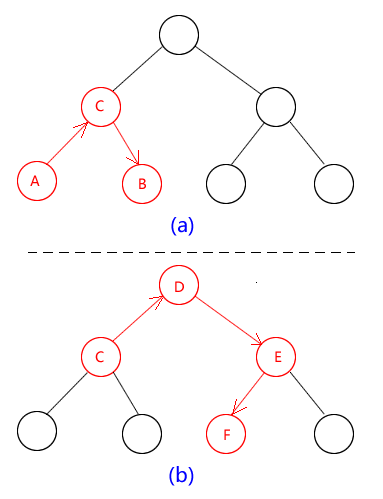图1

2、路径长度(Path Length)

3、结点的权重(Weight of Node)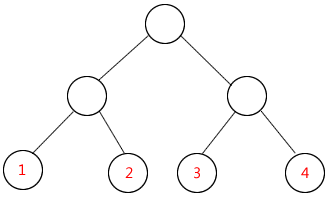图2

4、结点的带权(重)路径长度(Weight Path Length of Node):

5、树的带权(重)路径长度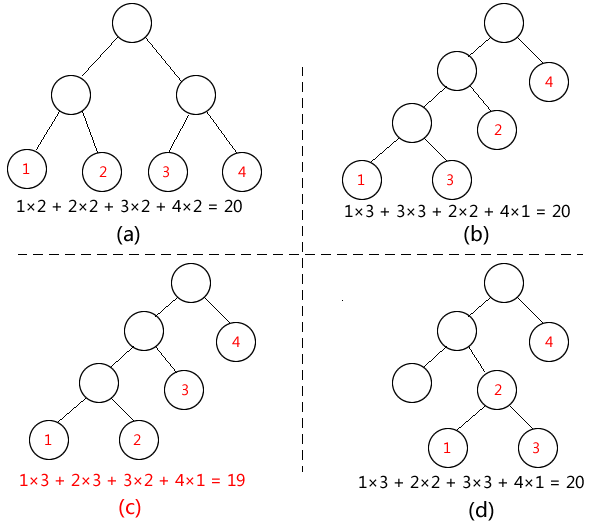1、在给定的带权叶节点中，找出权重最小的二个(通常为了方便，可以先将叶节点按权重从小到大先排好，这样只需要取前面二项即可)，然后添加一个临时节点作为这二个节点的父节点（其权重为这二个叶节点的权重之合）

2、将刚才处理过的二个节叶点去掉，然后把新增加的临时节点与剩下的叶节点放在一起做同样的处理，即：从新节点和叶节点的集合中，继续找到权重最小的二个，再继续增加新节点，做第1中的处理

3、重复以上过程，直到每个叶节点都处理完。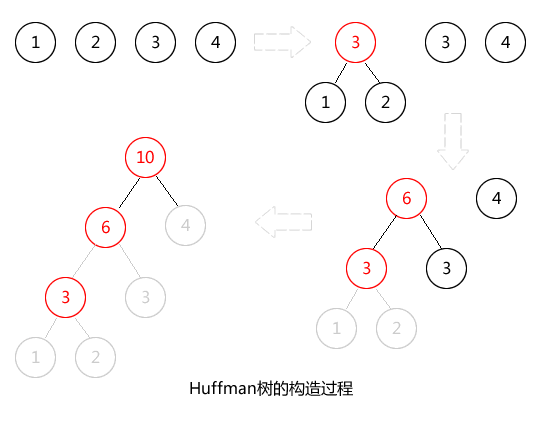c#的算法实现：

1、由二叉树的数学特性4知：

2、完全二叉树，可以方便的使用顺序存储（即用线性结构的数组或List<T>来存储）

Huffman树的节点类Node.cs:

```using System;
using System.Collections.Generic;
using System.Linq;
using System.Text;

namespace 哈夫曼树
{
public class Node
{
private int weight;//权重值
private int lChild;//左子节点的序号
private int rChild;//右子节点的序号
private int index;//本节点的序号

public int Weight
{
get { return weight; }
set { weight = value; }
}

public int LChild
{
get { return this.lChild; }
set { lChild = value; }
}

public int RChild
{
get { return this.rChild; }
set { rChild = value; }
}

public int Index
{
get { return this.index; }
set { index = value; }
}

public Node()
{
weight = 0;
lChild = -1;
rChild = -1;
index = -1;
}

public Node(int w, int lc, int rc, int p)
{
weight = w;
lChild = lc;
rChild = rc;
index = p;
}
}
}

```

HuffmanTree.cs(注：下面这段代码的Create算法在运行效率上也许并非最高的，但很容易理解)

```using System;
using System.Collections.Generic;
using System.Linq;
using System.Text;

namespace 哈夫曼树
{
public class HuffmanTree
{
private List<Node> _tmp;
private List<Node> _nodes;

public HuffmanTree(params int[] weights)
{
if (weights.Length < 2)
{
throw new Exception("叶节点不能少于2个!");
}

int n = weights.Length;

Array.Sort(weights);

//先生成叶子节点，并按weight从小到大排序
List<Node> lstLeafs = new List<Node>(n);
for (int i = 0; i < n; i++)
{
var node = new Node();
node.Weight = weights[i];
node.Index = i;
}

//创建临时节点容器
_tmp = new List<Node>(2 * n - 1);

//真正存放所有节点的容器
_nodes = new List<Node>(_tmp.Capacity);

}

/// <summary>
/// 构造Huffman树
/// </summary>
public void Create()
{
while (this._tmp.Count > 1)
{
var tmp = new Node(this._tmp.Weight + this._tmp.Weight, _tmp.Index, _tmp.Index, this._tmp.Max(c => c.Index) + 1);

//删除已经处理过的二个节点
this._tmp.RemoveAt(0);
this._tmp.RemoveAt(0);

//重新按权重值从小到大排序
this._tmp = this._tmp.OrderBy(c => c.Weight).ToList();
}
}

/// <summary>
/// 测试输出各节点的关键值(调试用)
/// </summary>
/// <returns></returns>
public override string ToString()
{
StringBuilder sb = new StringBuilder();
for (int i = 0; i < _nodes.Count; i++)
{
var n = _nodes[i];
sb.AppendLine("index:" + i + "，weight:" + n.Weight.ToString().PadLeft(2, ' ') + "，lChild_index:" + n.LChild.ToString().PadLeft(2, ' ') + "，rChild_index:" + n.RChild.ToString().PadLeft(2, ' '));
}
return sb.ToString();
}
}
}

```

```using System;

namespace 哈夫曼树
{
class Program
{
static void Main(string[] args)
{
HuffmanTree tree = new HuffmanTree(2,1,4,3);
tree.Create();

Console.WriteLine("最终树的节点值如下：");
Console.WriteLine(tree.ToString());
}
}
}

```

index:0，weight: 1，lChild_index:-1，rChild_index:-1
index:1，weight: 2，lChild_index:-1，rChild_index:-1
index:2，weight: 3，lChild_index:-1，rChild_index:-1
index:3，weight: 4，lChild_index:-1，rChild_index:-1
index:4，weight: 3，lChild_index: 0，rChild_index: 1
index:5，weight: 6，lChild_index: 2，rChild_index: 4
index:6，weight:10，lChild_index: 3，rChild_index: 5

输出结果也许并不直观，对照下面这张图就明白了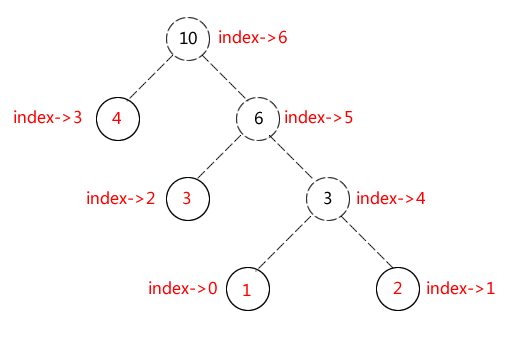A->00
B->01
C->10
D->11

A->0
B->10
C->111
D->110

00,00,00,00,01,01,01,10,10,11 (不算逗号共20位)

0,0,0,0,10,10,10,111,111,110 (不算逗号共19位)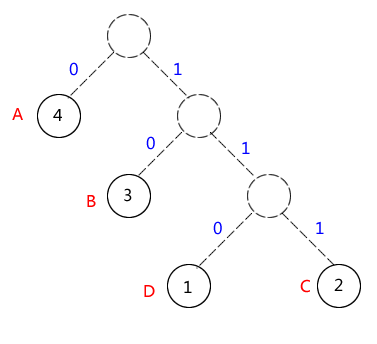OK，寻找哈夫曼编码的问题最终就转化成了哈夫曼树的构造问题，问题得到解决了。(学会了哈夫曼编码，也许我们能跟某些冰雪聪明的MM们玩点另类告白的小游戏，发一串数字过去，然后配一张图，看她懂不懂你的心意，如果她能成功解出背后的含义是ILOVEYOU，然后回发一串吉祥数字给你，那么...恭喜你！)

+ 关注